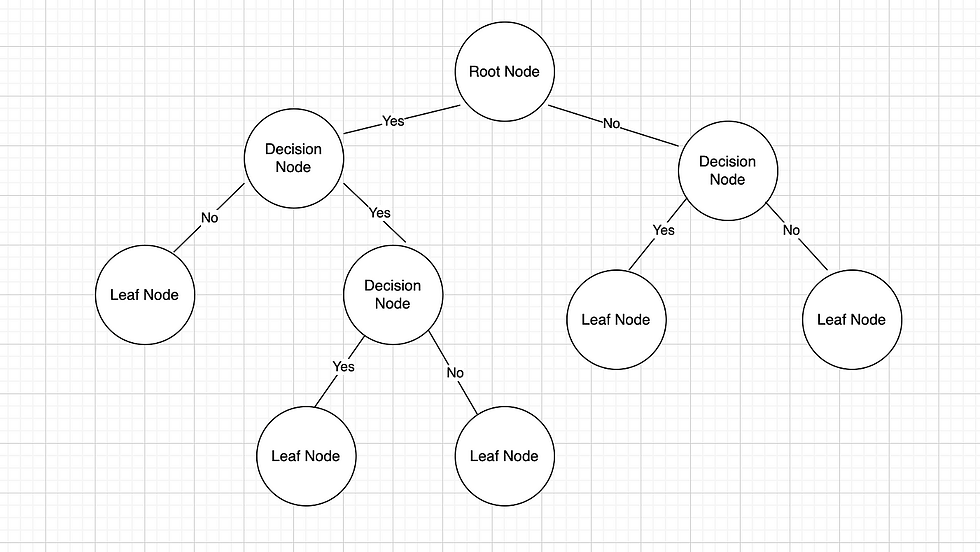top of pageSearch

# RandomForest Algorithm

Introduction :

Random Forest is one of the most important algorithm in machine learning, which comes under supervised learning. Supervised learning can be defined as the process where the algorithm will be trained and based on the trained data the predictions are made. Algorithms that comes under supervised learning are linear regression, logistic regression, Naive Bayes, support vector machine, decision tree, random forest, KNN, Neural networks.

Random Forest algorithm falls under tree models in ml. Tree-based models are built based on if-then rules to generate predictions from one or more decision trees.

Before learning random forest we need to have basic knowledge on decision tree. It is because random forest is a collection of multiple random decision tress. Random forest algorithm can be used for both regression and classification. Random forest can be used in different domains like fraud detection, healthcare, finance, marketing etc.,

Below is the link for basic understanding of decision tree.

Structure of Random Forest:

It has a hierarchical, tree structure, which consists of a root node, branches, internal nodes and leaf nodes. Multiple decision trees make a random forest.Classification : Let us imagine the result of the 5 random decision tree’s are as follows : 0,1,1,0,1. As we are calculating the classification majority of the individual decision trees result is considered. Here 1 have the majority and 1 is the result.

Regression : Let us imagine the result of the 5 random decision tree’s are as follows : 0,1,1,0,1. As we are calculating the regression mean or average of the individual trees result is considered. Here 0.6 is the result.

Let us consider an example of deciding whether a fruit is banana or apple or grape based on its shape, color, diameter.The first decision tree decides the fruits is an apple.The second decision tree decides the fruits is an apple.The third decision tree decides the fruits is a banana.

From the above three decision trees we found that two decision trees comes to an output of apple and one decision tree as banana. So the fruit will be classified as an apple.

Random forest algorithm is used to predict the employee performance in the below link:

We can observe that above trees have no impact on each other and they can run parallel using ensemble learning method. The subsets of the nodes in the tree is also selected by random forest algorithm only, which shows the scalability and feature importance of random forest algorithm.